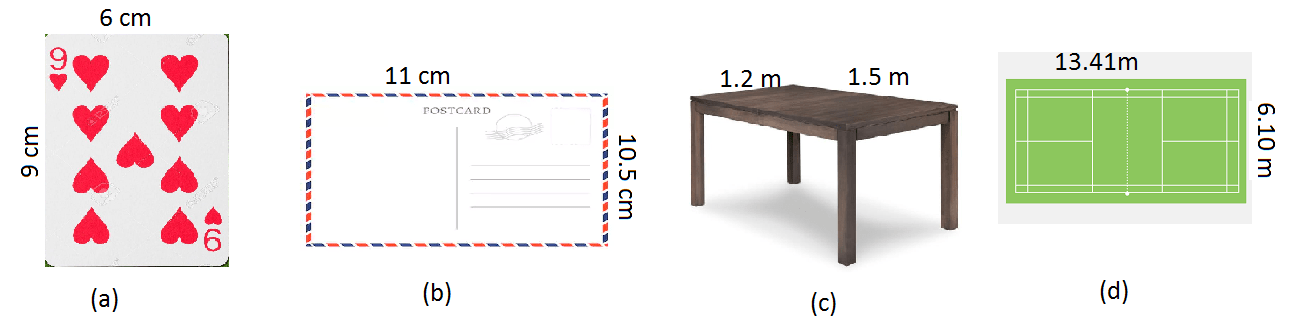# S. K. Gupta Anubhuti Gangal Class 5 Math Fifteenth Chapter Perimeter And Area Exercise 52

## EXERCISE 52

AREA: Area of a shape is the amount of space it covers.

(1) Copy and complete the table for the area rectangles:

(a) Length = 20 cm, Width = 8 cm

Solution: Area = (20 × 8) cm2 = 160 cm2

(b) Length = 14 km, Width = 10 km

Solution: Area = (14 × 10) km2 = 140 km2

(c) Length = 13 mm, Width = 3 mm

Solution: Area = (13 × 3) mm2 = 39 mm2

(d) Length = 12.5 m, Width = 10 m

Solution: Area = (12.5 × 10) m2 = 125 m2

(e) Length = 25 mm, Width = 60 mm

Solution: Area = (25 × 60) mm2 = 1500 mm2

(2) Calculate the area of each of the objects shown below:Solution: (a) The required area = (6 × 9) cm2 = 54 cm2

(b) The required area = (11 × 10.5) cm2 = 115.5 cm2

(c) The required area = (1.2 × 1.5) m2 = 1.8 m2

(d) The required area = (13.41 × 6.10) m2 = 81.801 m2

(3) Find the area of each of the rectangles described below:

(a) 4 cm by 3 cm

Solution: Area = (4 × 3) cm2 = 12 cm2

(b) 8 cm by 6 cm

Solution: Area = (8 × 6) cm2 = 48 cm2

(c) 20 cm 15 cm

Solution: Area = (20 × 15) cm2 = 300 cm2

(d) 28 cm by 21 cm

Solution: Area = (28 × 21) cm2 = 588 cm2

(e) Length = 1 m 75 cm, Width = 80 cm

Solution: Here, 1 m 75 cm = 175 cm

Area = (175 × 80) cm2 = 14000 cm2

(4) Find the area of a square whose one side is

(a) 3 cm

Ans: Area = (3)2 cm2 = 9 cm2

(b) 5 cm

Ans: Area = (5)2 cm2 = 25 cm2

(c) 13 cm

Ans: Area = (13)2 cm2 = 169 cm2

(d) 1.2 cm

Ans: Area = (1.2)2 cm2 = 1.44 cm2

(e) 2.5 cm

Ans: Area = (2.5)2 cm2 = 6.25 cm2

(f) 30 m

Ans: Area = (30)2 cm2 = 900 cm2

(g) 13.4 km

Ans: Area = (13.4)2 cm2 = 179.56 cm2

(h) 100 m

Ans: Area = (100)2 cm2 = 10000 cm2

(i) 12.16 m

Ans: Area = (12.16)2 cm2 = 147.8656 cm2

(5) Which square unit would you use to measure the area of:

(a) A page of this book

Ans: sq cm

(b) A stamp

Ans: sq mm

(c) The classroom floor

Ans: sq m

(d) Delhi

Ans: sq km

(e) The blackboard

Ans: sq m

(f) An insect’s wing

Ans: sq mm

(g) The Indian Ocean

Ans: sq km

(h) A sports field

Ans: sq m

(6) Choose the best estimate for the area. Write a, b or c.

(i) An envelope

Ans: (a) 200 cm2

(b) 200 mm2

(ii) A page of newspaper

Ans: (b) 2640 cm2

(7) Mary is making a quilt from square pieces of cloth. She fits 10 pieces along one side and 15 pieces along the other. How many pieces does she need?

Solution: The area of the cloth = (10 × 15) pieces = 150 pieces

Therefore, Mary needs a quilt from square of 150 pieces cloth.

(8) What is the area of the front of a watch that is 25 mm wide and 23 mm high?

Solution: The required area = (25 × 23) mm2 = 575 mm2

(9) Compare the areas of square 15 cm on each side and a rectangle 11 cm by 8 cm. Which figure has the greater area and by how much?

Solution: Area of the square = (15)2 cm2 = 225 cm2

Area of the rectangle = (11 × 8) cm2 = 88 cm2

(10) A rectangular field measures 400 m by 100 m and a square field 200 m by 200 m.

(i) Do the two fields have the same area?

Ans: Yes, the both fields have the same area.

(ii) Which field requires more fencing and by how much more?

Ans: Rectangular field, 200 meters more.

Updated: May 31, 2022 — 12:57 pm